In this post, we are going to learn how to apply a machine learning model for predictive analytics. We will create 5 models using different algorithms and test the results to compare which model gives the most accurate results. You can use this approach to compete on Kaggle or make predictions using your own datasets.

Dataset – For this experiment, we will use the birth_weight dataset from Delaware State Open Data Portal, which includes data from infants born in the period 2009-2016, including place of delivery (hospital/ birthing center/ home), gestation period (premature/ normal) and details about mother’s health conditions. You can download the data directly from the Open Data Link (https://data.delaware.gov/browse) or use the file provided with the code.

## Step 1 – Prepare the Workspace.

1. We clean up the memory of current R session, load some standard library packages (data.table, ggplot, sqldf, etc).
2. We load the dataset “Births.csv”.

## Step 2 – Data Exploration.

1. This step helps us understand the dataset – the range of values for variables, most common occurrences, etc. For our dataset, we look at a summary of birth years, birth weight and number of unique values.

2.  This is the point where we process for missing values and make a decision whether to ignore (entire column with large number of missing data), delete (very few records) or possibly replace it with median values. In this set however, there are no missing values that need to be processed.

3. Check how many unique values exist for each column.

## Step 3 – Test and Training Set

If you’ve ever competed on Kaggle, you will realize that the “training” set is the datafile used to create the machine learning model and the “test” set is the one where we use our model to predict the target variables.

In our case, we only have 1 file, so we will manually divide our set into 3 sets – one training set and one 2 test sets. (70% ,15%, 15% split) Why 2 test sets? Because it helps us better understand how the model reacts to new data. You can work with just one if you like. Just use one sequence command and stop with testdf command.

## Step 4 – Hypothesis Testing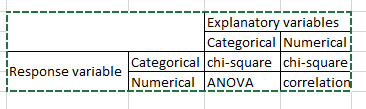statistical functions

In this step , we try to understand which predictors most affect our target variable using statistical functions such as ANOVA, chisquare, correlation, etc. The exact function you use can be determined using the table alongside.

Irrespective of which function we use, we assume the following hypothesis:
a) Ho (null hypothesis) – no relation exists. Ho is accepted if p-values if >= 0.05
b) Ha (alternate hypothesis) – relation exists. Ha is accepted if p-value < 0.05. If Ha is found true, then we conduct posthoc tests (for Anova and chisquare tests ONLY) to understand which sub-categories show significant differences in the relationship.

(1) Relation between birth_weight and mom’s_ethnicity exists since p-value < 0.05.

Using BONFERRONI adjustment and posthoc tests, we realize that mothers with “unknown” race are more likely to have babies with low birth weight, as compared to women of other races.

We also see this from the frequency table (below). Clearly only 70% of babies born to mothers of “unknown” race are of normal weight (2500 gms or above) compared to 92% babies from “other” race moms and 93% babies of White-race origins.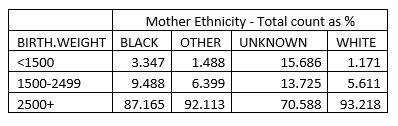mom ethnicity

(2) Relation between birth_weight and when prenatal_care started (first trimester, second, third or none) Although we see p-value < 0.05 Ha cannot be accepted because the posthoc tests do NOT show significant differences among prenatal care subsets.

(3) Relation between birth_weight and gestation period:

Posthoc tests show that babies in the groups POSTTERM 42+ WKS and TERM 37-41 WKS are similar and have higher birth weights than premature babies.
(4) We perform similar tests between birth_weight and multiple-babies (single, twins or triplets) and gender.

## Step 5 – Model Creation

We create 5 models:

• LDA (linear discriminant analysis) model with just 3 variables:
• LDA model with just 7 variables:
• Decision tree model:
• Model using Naïve Bayes theorem.
• Model using Neural Network theorem.

(1) Simple LDA model:

Model formula:

Make predictions with test1 file.

Examine how well the model performed.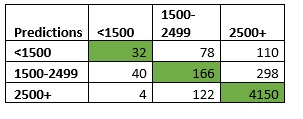lda_prediction-accuracy

From alongside table, we see that number of correct predictions (highlighted in green)

= (32+166+4150) / 5000

= 4348 / 50

= 0.8696

Thus, 86.96% predictions were correctly identified for test1! (Note, we will use the same process for checking all 5 models.)

Using a similar process, we get 88.4% correct predictions for test2.

#### (2) LDA model with just 7 variables:

Formula:

Make predictions for test1 and test2 files:

We get 87.6% correct predictions for test1 file and 88.57% correct for test2.

#### (3) Decision Tree Model

For the tree model, we first modify the birth weight variable to be treated as a “factor” rather than a string variable.

Model Formula:

Make predictions for test1 and test2 files:

We get 91.16% correct predictions for test1 file and 91.6% correct for test2. However, the sensitivity of this model is little low, since it has predicted that all babies will be of normal weight i.e “2500+” category. This is one of the disadvantages of tree models. If the target variable has a highly popular option which accounts for 80% or more records, then the model basically assigns everyone to it. (sort of brute force algorithm)

#### (4) Naive Bayes Theorem :

Model Formula:

Make predictions for test1 and test2 files:

Again we get model accuracy of 91.16% 91.6% respectively for test1 and test2 files. However, this model also suffers from a “brute-force” approach and has marked all babies with normal weight i.e “2500+” category. This reminds us that we must be careful about both accuracy and sensitivity of the model when applying an algorithm for forecasting purposes.

#### (5) Neural Net Algorithm Model :

Model Formula:

In the above formula, the “maxit” operation specifies a stop after maximum number of iterations, so that the program doesn’t go into an infinite loop trying to converge values. Since we have set the seed to 270, our formula converges after 330 iterations. With other “seed value” this number may be higher or lower.

Make predictions for test1 file:

Validation table (below) shows that total number of correct observations = 4592. Hence model forecast accuracy = 91.84%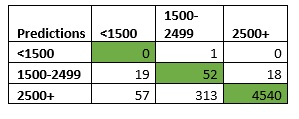nb_model_accuracy

Test with second file:

Thus, Neural Net models are accurate at 91.84% and 92.57% respectively for test1 and test2 respectively.

## Step 6 – Comparison of models

We take a quick look at how our models fared using a tabular comparison:  We conclude that neural network algorithm gives us the best accuracy and sensitivity.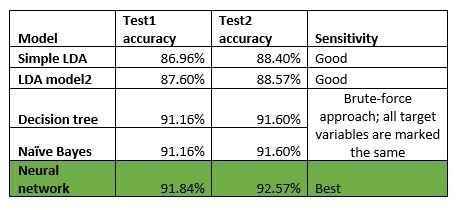compare data models

The code and datafiles for this tutorial are added to the New Projects page under “Jan” section. If you found this useful, please do share with your friends and colleagues. Feel free to share your thoughts and feedback in the comments section.